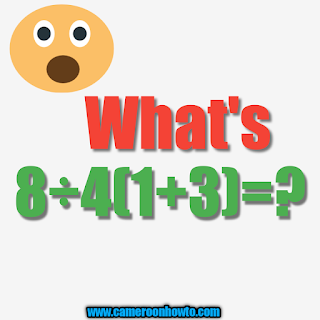# WHAT IS TRICKY MATH QUIZ 8÷4(1+ 3) = ? ANSWER# 8÷4(1+ 3) = ?

When we were in primary school, it was a sentence we use to say before solving a math Quiz like the one above.

explainations. Solving math quiz and puzzles can refresh your memory. When I first set the quiz and posted on Facebook, many people failed it. In facts , About 90% of those who solved it had wrong answers .

## Here is the Quiz: WHAT IS THE ANSWER TO THE TRICKY MATH QUIZ 8÷4(1+ 3) = ?

The correct answer would be 8. I am going to solve using PEMDAS.

## Solution

I will solve this Quiz today using PEMDAS.
PEMDAS is a mathematical acronym which stands for Parentheses, Exponent, Multiplication, Division, Addition and Subtraction.

### Get lettle insight about PEMDAS below.

P=Parenthesis ()
E=Exponent
M=Multiplication
D=Division
S=Subtraction.

With PEMDAS, to solve algebraic expressions, it is further grouped into four important stages in order of merit.
First: Parenthesis () or Bracket [].
Second: Exponents
Third: multiplication and Division.
This stage is very important to note because this is where the left to right rule, if not applied may give different answers.check example 1 above.

So, 8÷4(1+3)= ?
first thing is to solve what is in the  Parenthesis.
1+3=4.

Now, I will rearrange the quiz and simplify as thus:
8÷4(4)=?
=>8÷4×4=?
=>2×4=?
=> 8 =?.

Therefore the final answer to the TRICKY MATH QUIZ
8÷4(1+ 3) = ? is 8

Conclusion
Solving with PEMDAS will give you same asame answer with someone who solved applying BODMAS.